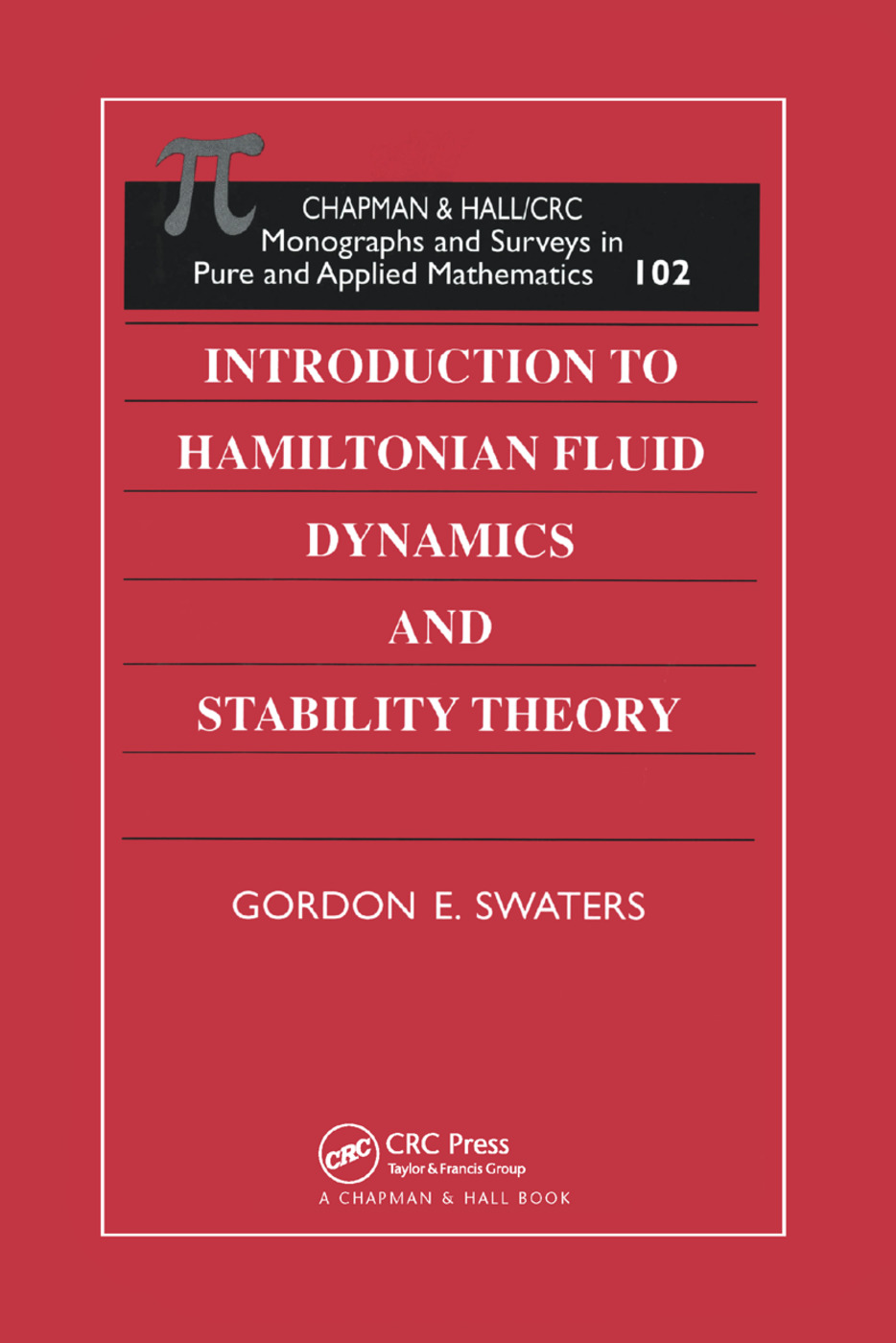# Introduction to Hamiltonian Fluid Dynamics and Stability Theory

## 1st Edition

Chapman and Hall/CRC

288 pages

Paperback: 9780367399405
pub: 2019-10-07
SAVE ~\$14.99
\$74.95
\$59.96
x
Hardback: 9781584880233
pub: 1999-09-29
SAVE ~\$39.00
\$195.00
\$156.00
x
eBook (VitalSource) : 9780203750087
pub: 2019-01-22
from \$37.48

FREE Standard Shipping!

### Description

Hamiltonian fluid dynamics and stability theory work hand-in-hand in a variety of engineering, physics, and physical science fields. Until now, however, no single reference addressed and provided background in both of these closely linked subjects. Introduction to Hamiltonian Fluid Dynamics and Stability Theory does just that-offers a comprehensive introduction to Hamiltonian fluid dynamics and describes aspects of hydrodynamic stability theory within the context of the Hamiltonian formalism.

The author uses the example of the nonlinear pendulum-giving a thorough linear and nonlinear stability analysis of its equilibrium solutions-to introduce many of the ideas associated with the mathematical argument required in infinite dimensional Hamiltonian theory needed for fluid mechanics. He examines Andrews' Theorem, derives and develops the Charney-Hasegawa-Mima (CMH) equation, presents an account of the Hamiltonian structure of the Korteweg-de Vries (KdV) equation, and discusses the stability theory associated with the KdV soliton.

The book's tutorial approach and plentiful exercises combine with its thorough presentations of both subjects to make Introduction to Hamiltonian Fluid Dynamics and Stability Theory an ideal reference, self-study text, and upper level course book.

Introduction The Nonlinear Pendulum Model Formulation Canonical Hamiltonian Formulation Least Action Principle Symplectic Hamiltonian Formulation Mathematical Properties of the J Matrix Poisson Bracket Formulation Steady Solutions of a Canonical Hamiltonian System Linear Stability of a Steady Solution Nonlinear Stability of a Steady Solution The Two Dimensional Euler Equations Vorticity Equation Formulation Hamiltonian Structure for Partial Differential Equations Hamiltonian Structure of the Euler Equations Reduction of the Canonical Poisson Bracket Casimir Functionals and Noether's Theorem Exercises Stability of Steady Euler Flows Steady Solutions of the Vorticity Equation Linear Stability Problem Normal Mode Equations for Parallel Shear Flows Linear Stability Theorems Nonlinear Stability Theorems Andrews' Theorem Flows with Special Symmetries Exercises The Charney-Hasegawa-Mima Equation A Derivation of the CHM Equation Hamiltonian Structure Steady Solutions Stability of Steady Solutions Steadily-Travelling Solutions Exercises The KdV Equation A Derivation of the KdV Equation Hamiltonian Structure Periodic and Soliton Solutions Variational Principles Linear Stability Nonlinear Stability Exercises

Swaters, Gordon E